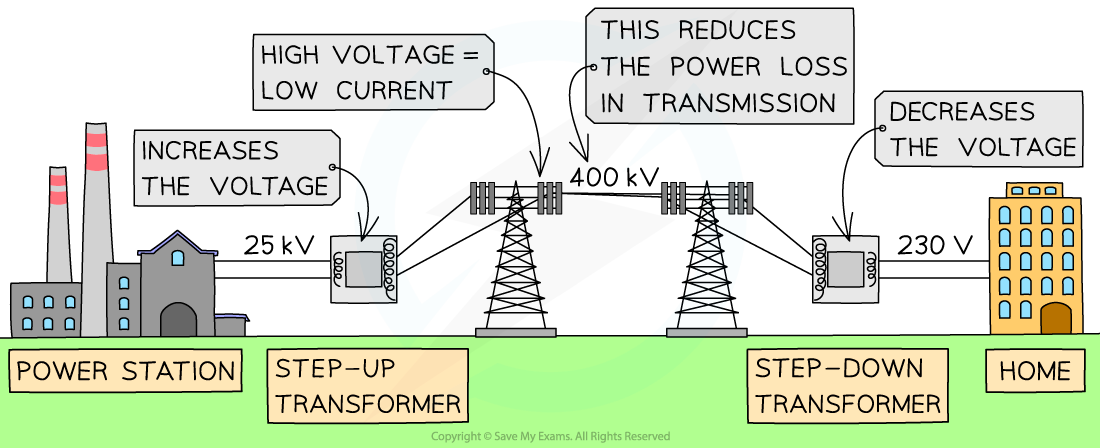# IB DP Physics: HL复习笔记11.2.5 AC Electrical Power Distribution

### AC Electrical Power Distribution

• Energy losses due to the heating of transmission lines in national power grids are significant
• This is because the electrical energy is transmitted across long distances from power stations to buildings
• Inefficiencies in a transformer appear not from just the core, but also in the wires
• The coils of wire have resistance
• This causes heat energy to be lost from the current flowing through the coils
• The larger the current, the greater the amount of heat energy lost
• In the core, the inefficiencies appear from:
• Induced eddy currents
• The reversal of magnetism
• Poor insulation between the primary and secondary coil
• Ways to reduce energy loss in a transformer are:
• Making the core from soft iron or iron alloys to allow easy magnetisation and demagnetisation and reduce hysteresis loss
• Laminating the core
• Using thick wires, especially in the secondary coil of step-down transformers
• Using a core that allows all the flux due to the primary coil to be linked to the secondary coil
• Power losses from the current are calculated using the equation:

P = I2R

• Where:
• P = power (W)
• I = current (A)
• R = resistance (Ω)
• The equation shows that:
• P ∝ I2
• This means doubling the current produces four times the power loss
• Therefore, step-up transformers are used to increase the voltage which decreases the current through transmission lines
• This reduces the overall heat energy lost in the wires during transmission
• A step-down transformer is then used to decrease the voltage to that required in homes and buildingsThe use of step-up and step-down transformers in the National Grid

#### Worked Example

A current of 2500 A is transmitted through 150 km of cables. The resistance of the transmission cable is 0.15 Ω per km.

Calculate the power wasted.

Step 1: List the known quantities

• Current, I = 2500 A
• Length of cables, L = 150 km = 150 × 103 m
• Resistance of the cables, R = 0.15 Ω km-1

Step 2: Write out the power equation

P = I2R

Step 3: Determine the total resistance, R

R = Resistance of the wires × Length of wires

R = 0.15 × 150

Step 4: Substitute values into the power equation

Power lost = (2500)2 × (0.15 × 150) = 141 MW